# Dice Probability Calculator

Use this dice odds calculator to easily calculate any type of dice roll probability: sum of two dice, sum of multiple dice, getting a value greater than or less than on a given throw of N dice, and so on. Different types of dice are supported: from four-sided, six-sided, all the way to 20-sided (D4, D6, D8, D10, D12, and D20) so that success probabilities and dice odds in popular dice games can be computed.

Share calculator:

Embed this tool:
get code

## Using the dice probability calculator

The tool can be used to compute dice probabilities for any type of game of chance or probability problem as used in teaching basic statistical concepts such as sample space and p-values. It supports the classic scenario of computing probabilities of the sum of two six-sided dice, but also supports 4-sided, 8-sided, 10-sided, 12-sided, and 20-sided dice. In the classic problem two dice are thrown, but with this dice calculator you can also explore it with three or more dice.

The calculator can output, depending on the choice of probability calculation, the probability of:

• throwing an exact sum of two or more dice
• a sum less than or greater than or equal to a given number
• at least one die having a face equal to a given number
• all dice having rolling a value equal to a given number
• at least one die rolling a value less than or equal, or greater than or equal to a number
• all dice rolling a value less than or equal, or greater than or equal to a number

With such versatility you can calculate dice probabilities for most games of chance such as Craps, Backgammon, Dungeons & Dragons (D&D), Balut, Dice 10000, Diceball!, Dudo, Elder Sign, Kismet, Yahtzee, Bunco, and many more.

## Two (6-sided) dice probabilities

The classic case of exploring dice throw probabilities (dice rolling odds) is to estimate the chance of landing a given sum on the faces of two six-sided dice. In this example, two dice are thrown together and one records their face values, and computes their sum. Each die is a cube with six faces with the numbers from one to six printed on each side. One throws two dice and the value of a roll is whatever number faces up once the die settles in place.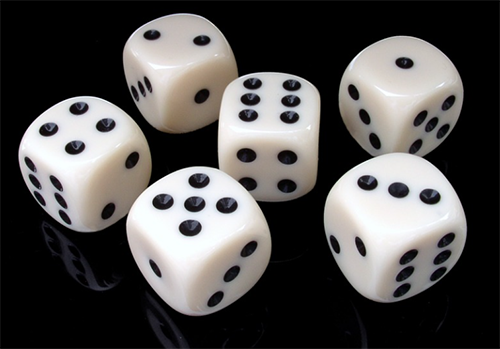The image above shows six such dice each with a different face up. The question is: what is the probability of rolling a given sum with two six-sided dice?. The dice are assumed to be fair (unbiased), meaning each side has equal probability of turning up. Also, the outcome of each roll is independent of rolls preceding or succeeding it. A series of fair dice rolls can be modelled as independent events.

### Sample space of the two dice problem

In essence, one needs to first estimate the size of the set of all possible outcomes of the dice throw known as the sample space, and then figure out how many of these result in the desired sum. To solve this problem, one needs to calculate every possibility which might turn up which amounts to estimating how many possible permutations there are in total.

For two 6-sided dice there are exactly 6^2 = 6 · 6 = 36 possible permutations with repetition (selecting two numbers from a set of six).

### Calculating two (6-sided) dice probability

Knowing the sample space means now we need only compute how many possible ways there are for the dice to result in the sum of interest. For example, if the sum of interest is 12, there is just a single dice permutation which results in such a sum - it only happens if both dice thrown roll a six (double sixes, a.k.a. 'boxcars'). The chance to roll a twelve is then 1 out of 36 = 1 / 36 = 0.02777 or 2.77%. It is also the chance of rolling double ones (a.k.a. 'snake eyes').

If the question is what is the chance of throwing a seven with two dice, then one should consider all possible permutations in which a seven turns up. A seven can be the sum of (6 and 1), (5 and 2), (4 and 3) for a total of three possible permutations, and then we need to consider them in the other possible way, namely (1 and 6), (2 and 5), (3 and 4), for a total of six permutations. So we have six chances out of thirty six, meaning the probability of throwing exactly seven is 6/36 = 1/6 = 0.1666 = 16.66%.

### Two dice probability chart

Doing the calculations for all possible outcomes between two and twelve for the sum of two dice rolls one arrives at the following chart of dice probabilities and dice odds. It is for two dice rolled simultaneously or one after another (classic 6-sided dice):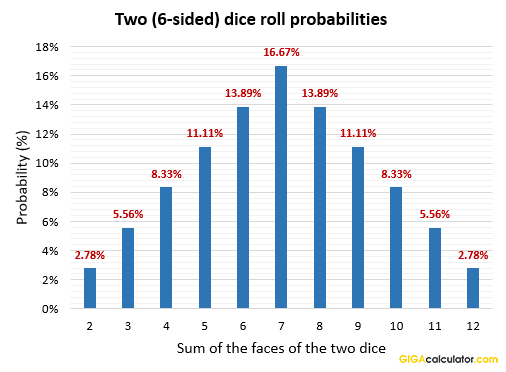If two dice are thrown together, the odds of getting a seven are the highest at 6/36, followed by six and eight with equal odds of 5/36 (13.89%), then five and nine with odds of 4/36 (11.11%), and so on. Here is the same information in table form:

Sum of two (6-sided) dice probabilities
Roll a sum ofProbability (Odds)
2 2.778% (1/36)
3 5.556% (2/36)
4 8.333% (3/36)
5 11.111% (4/36)
6 13.889% (5/36)
7 16.667% (6/36)
8 13.889% (5/36)
9 11.111% (4/36)
10 8.333% (3/36)
11 5.556% (2/36)
12 2.778% (1/36)

## Dice probability formula

The probability (P) of rolling a given sum (p) by throwing any number of dice (n) with a given number of sides or faces (s) can be calculated using the general formula as provided by Uspensky 1937 :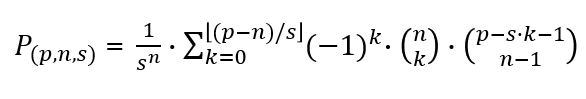The brackets ⌊ ... ⌋ in the upper limit of the sigma operator denote the floor mathematical function. The round brackets with two numbers one on top of the other denote combinations without repetition. It is read as "n choose k" and its computation uses the factorial function. This makes it somewhat difficult to compute the equation by hand so a dice probability calculator comes in super handy even with a small number of dice.

The formula can be used to produce dice probability distribution charts for any type and number of dice, and dice rolls. With a larger number of dice the distribution converges to the normal distribution in accordance with the Central Limit Theorem . Calculating the probability of rolling greater than or less than a certain sum simply entails summing the probabilities of all possible outcomes greater than the sum of interest.

## Probabilities with a single die roll

The probability of rolling any given number with a single die on a single roll is equal to one divided by the number of die faces. For example, with a 20-sided die, the odds of rolling 20 is 1/20 or a 5% chance. With a classic six-sided die the probability of seeing any particular face is 16.667% or chance odds of 1/6. As you can see, single die roll probabilities require only simple division to calculate.

## Probability of rolling a certain number with n dice throws

If there is more than one throw possible, e.g. if throwing two dice simultaneously or one after another, the probability of rolling a given number with at least one of the throws increases. However, it never reaches 100%! For example, if one needs a six and has six throws of a D6 die, the probability of rolling a six is not 16.667% · 6 = 100%.

A clever way to easily calculate it is to first raise the probability of not getting a six on each throw to the power of the number of throws possible. The probability of not rolling a six is (6-1) / 6 = 5/6 or 0.8333. With six dice, we have 5/6^6 = 5/6 · 5/6 · 5/6 · 5/6 · 5/6 · 5/6 = 0.3349. This means that the probability of throwing at least one six with six tries is 1 - 0.3349 = 0.6651 or 66.51% giving odds of roughly 2/3. In effect, the multiplication in the denominator calculates the sample space whereas the multiplication in the numerator computes the number of unfavourable outcomes in the sample space. We have 5^5 = 15,625 possibilities for not seeing a single six out of 6^6 = 46,656 total possibilities.

Here is a chart of the probabilities of getting at least one six with increasing number of dice rolls: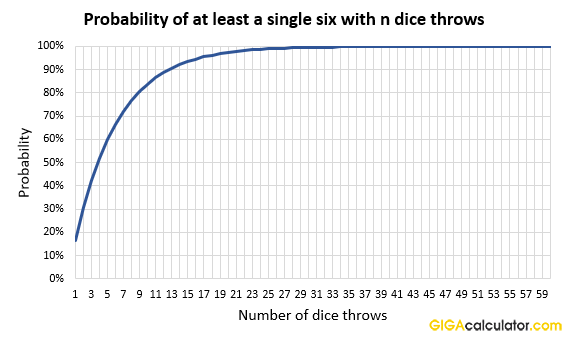While the probability increases slowly with each subsequent dice throw, it never actually reaches 100%.

## Probability of rolling a certain number on all n dice

In contrast to the probability of throwing a certain number by having n tries (either throwing n dice together or throwing a die n times), the probability of rolling the same face value on a number of dice decreases substantially the more dice there are. The probability never reaches zero, but it can be considered practically nil after a certain number of dice. Here is a graph with these probabilities: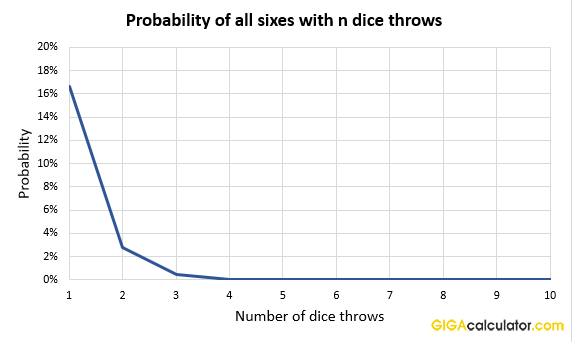With just ten dice throws, the probability of rolling a six on all is a mere 0.000002%, and the chance only decreases further when more dice throws are added.

## Examples

Here are a few more examples for the odds in different games with dice.

### What are the odds of throwing more than 9 at craps?

Craps is played with two six-sided dice. To calculate the odds of rolling 9 or more we need to use the dice probability formula above and compute the probabilities for all possible outcomes of throwing the two dice: 9, 10, 11, and 12, then sum them up. Using the table above we can see the odds are 4/36, 3/36, 2/36, and 1/36 respectively. Summing them up (you can use our fractions calculator for this task) results in odds of 10/36 (10 to 36), or a probability of 0.2778 (27.78%).

### What are the odds of rolling 38 or more in D&D?

Dice play an important role in the tabletop game Dungeons and Dragons (DnD). Sometimes one needs to roll a high number to have a chance at performing a given action such as successfully casting a spell. What is the probability of rolling 38 or more given that one can throw two 20-sided dice? Using the equation for the sum of n dice above, we can compute the probability of getting exactly 38, 39, and 40 to be 0.75%, 0.5%, and 0.25%. Summing these up, we get that the chance to roll 38 or higher in D&D, is 0.75% + 0.5% + 0.25% = 1.5% (or odds of 1 out of 66.7).